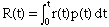Name: Brian Who is asking: Student Level: Secondary Question: Suppose that t months from now an oil well will be producing crude oil at the rate of r(t), not a constant, barrels per month and that the price of crude oil will be p(t), not a constant, dollars per barrel. Assume that the oil is sold as soon as it is extracted from the ground. Find an expression for the total revenue from the oil well, R(t). A certain oil well that currently yields 400 barrels of crude oil a month will run dry in 2 years. The price of crude oil is currently \$18 per barrel and is expected to rise at a constant rate of 3 cents per barrel per month. What will be the total revenue from this well? {Hint: Model the degraded production rate with the equation: r(t) = (A-Bt)e0.04t} Thanks! Hi Brain, If the well is producing at the rate of r(t) barrels/month and the price is p(t) dollars/barrel then the revenue function is r(t)*p(t) dollars/month. If you want the total revenue R(t) from time zero to time t months then you need to "add" the revenue for each month. Since the revenue function r(t) is continuous, "adding" the instantaneous revenues is accomplished by integration. That isFor part 2. use the fact that r(0) = 400 barrels/month and r(48) = 0 barrels/month to find A and B. Use a linear function to model the price function p(t) and then apply the expression above. Cheers, Harley Go to Math Central